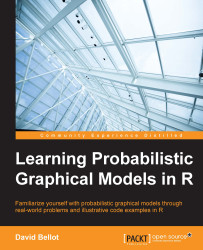•#### Learning Probabilistic Graphical Models in R#### Overview of this book

Probabilistic graphical models (PGM, also known as graphical models) are a marriage between probability theory and graph theory. Generally, PGMs use a graph-based representation. Two branches of graphical representations of distributions are commonly used, namely Bayesian networks and Markov networks. R has many packages to implement graphical models. We’ll start by showing you how to transform a classical statistical model into a modern PGM and then look at how to do exact inference in graphical models. Proceeding, we’ll introduce you to many modern R packages that will help you to perform inference on the models. We will then run a Bayesian linear regression and you’ll see the advantage of going probabilistic when you want to do prediction. Next, you’ll master using R packages and implementing its techniques. Finally, you’ll be presented with machine learning applications that have a direct impact in many fields. Here, we’ll cover clustering and the discovery of hidden information in big data, as well as two important methods, PCA and ICA, to reduce the size of big problems.
Learning Probabilistic Graphical Models in RCreditswww.PacktPub.comPrefaceFree Chapter
Probabilistic ReasoningExact InferenceLearning ParametersBayesian Modeling – Basic ModelsApproximate InferenceBayesian Modeling – Linear ModelsProbabilistic Mixture ModelsAppendixIndex## Basic sampling algorithms

We start our study of sampling algorithms by looking at some basic algorithms that can be used as subroutines in more advanced schemes. In all the algorithms from this book, we assume that we can generate random numbers uniformly from the interval [0,1]. The problem of generating random numbers on a computer is complex and vast. In most cases, random numbers will be generated by a deterministic algorithm, and they are called pseudo-random numbers. They are not random, but their distribution and properties are close enough to a real random generator that they can be used as real random numbers. Pseudo-random number generators are usually based on the estimation of chaotic functions that are extremely sensitive to their initial conditions, which are called the seed. Changing the value can generate a completely different sequence of numbers, even if the seed value is just a little bit different from the previous one. Nowadays, we also have electronic devices that can...